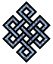#fail2ban bad ip database: ip 123.31.28.171

### | ip database | live view | stats | report | help | api key:

 ip: 123.31.28.171 hostname: static.vnpt.vn country:[VN] Vietnam first reported: 15.11.2018 07:09.57 GMT+0200 last reported: 13.04.2019 12:29.28 GMT+0200 time period: 149d 04h 19m 31s total reports: 11 reported by: 2 host(s) filter(s): ssh (11) tor exit node no badips.com db Lookup## port scan of '123.31.28.171':

[-hide]
```# Nmap 6.40 scan initiated Thu Nov 15 07:10:03 2018 as: /usr/bin/nmap -sU -sS -O 123.31.28.171
Nmap scan report for static.vnpt.vn (123.31.28.171)
Host is up (0.19s latency).
Not shown: 1000 open|filtered ports, 988 filtered ports
PORT     STATE  SERVICE
22/tcp   open   ssh
80/tcp   open   http
443/tcp  open   https
3000/tcp open   ppp
3001/tcp closed nessus
3306/tcp open   mysql
3389/tcp open   ms-wbt-server
5432/tcp closed postgresql
6969/tcp closed acmsoda
7777/tcp closed cbt
8080/tcp open   http-proxy
8888/tcp open   sun-answerbook
No exact OS matches for host (If you know what OS is running on it, see http://nmap.org/submit/ ).
TCP/IP fingerprint:
OS:SCAN(V=6.40%E=4%D=11/15%OT=22%CT=3001%CU=%PV=N%G=Y%TM=5BED0DE5%P=x86_64-
OS:pc-linux-gnu)SEQ(SP=104%GCD=1%ISR=10B%TI=Z%TS=A)SEQ(SP=104%GCD=1%ISR=10B
OS:%TI=Z%CI=RD%TS=A)OPS(O1=M5B4ST11NW7%O2=M5B4ST11NW7%O3=M5B4NNT11NW7%O4=M5
OS:B4ST11NW7%O5=M5B4ST11NW7%O6=M5B4ST11)WIN(W1=7120%W2=7120%W3=7120%W4=7120
OS:%W5=7120%W6=7120)ECN(R=Y%DF=Y%TG=40%W=7210%O=M5B4NNSNW7%CC=Y%Q=)T1(R=Y%D
OS:F=Y%TG=40%S=O%A=S+%F=AS%RD=0%Q=)T2(R=N)T3(R=N)T4(R=Y%DF=Y%TG=40%W=0%S=A%
OS:A=Z%F=R%O=%RD=0%Q=)T5(R=Y%DF=Y%TG=40%W=0%S=Z%A=S+%F=AR%O=%RD=0%Q=)T6(R=Y
OS:%DF=Y%TG=40%W=0%S=A%A=Z%F=R%O=%RD=0%Q=)T7(R=Y%DF=Y%TG=40%W=0%S=Z%A=S+%F=
OS:AR%O=%RD=0%Q=)U1(R=N)IE(R=Y%DFI=N%TG=40%CD=S)

OS detection performed. Please report any incorrect results at http://nmap.org/submit/ .
# Nmap done at Thu Nov 15 07:10:45 2018 -- 1 IP address (1 host up) scanned in 42.97 seconds
```
```Σ = 113 | Δt = 0.0048651695251465s
```